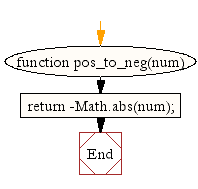# JavaScript: Convert a positive number to negative number

## JavaScript Math: Exercise-29 with Solution

Write a JavaScript function to convert a positive number to a negative number.

Test Data :
console.log(pos_to_neg(15));
-15

Sample Solution:-

HTML Code:

``````<!DOCTYPE html>
<html>
<meta charset="utf-8">
<title>JavaScript function to convert a positive number to negative number.</title>
<body>

</body>
</html>
```
```

JavaScript Code:

``````function pos_to_neg(num)
{
return -Math.abs(num);
}

console.log(pos_to_neg(15));
```
```

Sample Output:

```-15
```

Flowchart:Live Demo:

See the Pen javascript-math-exercise-29 by w3resource (@w3resource) on CodePen.

Improve this sample solution and post your code through Disqus

What is the difficulty level of this exercise?

Test your Programming skills with w3resource's quiz.

﻿